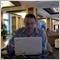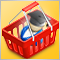# Automatic validation Invalid Order send error 131267

```Hi Gents, I have the following code  it works perfect on my EA  tester

but when it comes to automatic validation  it fails

int start()

{

//----

int TCC = 0;

for(int ii = OrdersTotal()-1; ii >= 0; ii--) {

if(OrderSymbol() != Symbol() || OrderMagicNumber() != magic_number) continue;

TCC++; double gh= OrderProfit(); //double  ghhh=OrderOpenPrice();int TMMM=OrderOpenTime();

//if (OrderType()==OP_SELL)double kkkk=OrderProfit(),uuuu=OrderLots();

}

if (Bars < 100) { Print ("Bars less than 100."); return (0);

double opachD1=iCustom(NULL,60,"\\Examples\Heiken_Ashi_Smoothed_TF.ex4",2,4,2,1,0,1);

double clachD1=iCustom(NULL,60,"\\Examples\Heiken_Ashi_Smoothed_TF.ex4",2,4,2,1,1,0);

double opachDd1=iCustom(NULL,1440,"\\Examples\Heiken_Ashi_Smoothed_TF.ex4",2,4,2,1,0,1);

double clachDd1=iCustom(NULL,1440,"\\Examples\Heiken_Ashi_Smoothed_TF.ex4",2,4,2,1,1,0);

}

total = OrdersTotal();

if ((total == 0) && (level == 0))

{

//  int trend = iMA(NULL,1,25,0,MODE_LWMA,PRICE_CLOSE,0);

RefreshRates();

//   if ((trend - Ask < 0) && (trend - Bid < 0))

if ((iClose(NULL,5,1)== iOpen(NULL,5,1) && iClose(NULL,5,0)>iOpen(NULL,5,0) && TCC<1)||(iOpen(NULL,60,0)< iClose(NULL,60,0) && iOpen(NULL,60,0)<clachD1 && iClose(NULL,60,0)>opachD1   && opachD1>clachD1 && TCC<1  )  )

{

level = 1;

return (0);

}

//   if ((trend - Ask > 0) && (trend - Bid > 0))

if ((iClose(NULL,5,1)== iOpen(NULL,5,1) && iClose(NULL,5,0)<iOpen(NULL,5,0) && iHigh(NULL,5,1)>iHigh(NULL,5,2)  && TCC<1)||(iOpen(NULL,60,0)> iClose(NULL,60,0) && iOpen(NULL,60,0)>clachD1 && iClose(NULL,60,0)<opachD1 && opachD1<clachD1 && TCC<1    )  )

{

ticket[level] = Sell(level);

level = 1;

return (0);

}

else {return (0);}

// this is the initial buy or sell position

}

if ((total>=1) && (level >= 1) && (total <=7))

{

if(OrderType() == OP_BUY) pips = (MarketInfo(OrderSymbol(), MODE_BID) - OrderOpenPrice())/MarketInfo(OrderSymbol(),MODE_POINT);

if(OrderType() == OP_SELL) pips= (OrderOpenPrice() - MarketInfo(OrderSymbol(),MODE_ASK))/MarketInfo(OrderSymbol(),MODE_POINT);

double profit = AccountProfit();

// if the order gives a \$1 profit, close it/them...

// or if the orderS give a \$25 loss, close them...

if (((total == 1) && (profit>2)) || ((total == 2) && (profit>2))  || ((total == 3) && (profit>5)) || ((total == 4) && (profit>5)))// ||((total == 5) && (profit> 5)) || ((total == 6) && (profit>5)) || ((total == 7) && (profit>0 )))//|| ((total == 8) && (profit >0))  )

{

CloseAll();

level = 0;P=0;

return (0);

}

// while the total orders are still below level 5, go on with the hedge...

else if (level != 4)

{

if(OrderType() == OP_BUY) pips = (MarketInfo(OrderSymbol(), MODE_BID) - OrderOpenPrice())/MarketInfo(OrderSymbol(),MODE_POINT);

if(OrderType() == OP_SELL) pips= (OrderOpenPrice() - MarketInfo(OrderSymbol(),MODE_ASK))/MarketInfo(OrderSymbol(),MODE_POINT);

if (pips <= -1500-P)

{

if(OrderType() == OP_BUY && OrderLots()<SymbolInfoDouble(Symbol(),SYMBOL_VOLUME_MAX) && OrderLots()>SymbolInfoDouble(Symbol(),SYMBOL_VOLUME_MIN))

{

ticket[level] = Sell(level);

level = level + 1;P=P+0;

return (0);

}

if(OrderType() == OP_SELL && OrderLots()<SymbolInfoDouble(Symbol(),SYMBOL_VOLUME_MAX))

{

level = level + 1; P=P+0;

return (0);

}

}

}

else

{if (OrderType()==OP_SELL && total>3) {OrderModify(OrderTicket(), OrderOpenPrice(), OrderOpenPrice()+500*Point, 0, 0, DarkOrange);

OrderModify(OrderTicket(), OrderOpenPrice(),0, OrderOpenPrice()+1000*Point,  0, DarkOrange);}

if (OrderType()==OP_BUY && total>3){ OrderModify(OrderTicket(), OrderOpenPrice(), OrderOpenPrice()-500*Point, 0, 0, DarkOrange);

OrderModify(OrderTicket(), OrderOpenPrice(),0, OrderOpenPrice()-1000*Point,  0, DarkOrange);}  }

{ObjectCreate("ObjName1", OBJ_LABEL, 0, 0, 0);

ObjectSetText("ObjName1",MarketInfo(Symbol(),MODE_LOTSTEP)+"    "+MarketInfo(Symbol(),MODE_MINLOT) ,14, "Verdana", Yellow);

ObjectSet("ObjName1", OBJPROP_CORNER, 0);

ObjectSet("ObjName1", OBJPROP_XDISTANCE, 400);

ObjectSet("ObjName1", OBJPROP_YDISTANCE, 30);}

}

//----

return(0);

}

//+------------------------------------------------------------------+

void CloseAll ()

{

int totalorders = OrdersTotal();

int cnt = 0;

for (cnt = totalorders-1 ; cnt >=0 ; cnt--)

{

if(Symbol()!=OrderSymbol()) RefreshRates();

if(OrderType()>OP_SELL) OrderDelete(OrderTicket());

}

// return (0);

}

{

double Lots  = {0.02 , 0.04 , 0.08,0.16 };//, 0.3,0.4,0.6};// , 0.80 , 0.40 , 0.50 };

return (ticket_num);

}

int Sell (int nth_level)

{

double Lots  = {0.02 , 0.04, 0.08,0.16 };//, 0.3, 0.4, 0.6 };//, 0.25, 0.40 , 0.50 };

int ticket_num = OrderSend (Symbol(),OP_SELL,Lots[nth_level],Bid,3,0,0,"",12345,0,Red);

return (ticket_num);

}

GENTS  I WOULD REALLY APPRECIATE  iF you help me  why I did get fail problem in automatic  validation

THANKS ```Moderator
114398267

Sergey Golubev:

Ok it's done  do you have an idea  why  I keep getting  error message 131 order send failure

backtesting  is OKModerator
114398

Ok it's done  do you have an idea  why  I keep getting  error message 131 order send failure

backtesting  is OK

I am not a coder sorry (I just hope the members will help).38753

Ok it's done  do you have an idea  why  I keep getting  error message 131 order send failure

backtesting  is OK

Did you check the meaning of error 131 ?The checks a trading robot must pass before publication in the Market
• 2016.08.01
• MetaQuotes Software Corp.
• www.mql5.com
Before any product is published in the Market, it must undergo compulsory preliminary checks in order to ensure a uniform quality standard. This article considers the most frequent errors made by developers in their technical indicators and trading robots. An also shows how to self-test a product before sending it to the Market.267

Alain Verleyen:

Did you check the meaning of error 131 ?

yes  I already checked it  it deals with invalid  Volume  ,  my issue is the following my EA works fine  in back testing no errors in demo as well  but when i tried to  sell  my EA  it should pass through automatic validation  and  suddenly this error pops up   i can't figure out  the origin

I  have  tried  to investigate on  min, max lot size LOtsize ans step LOT i got the followings  100000 ( standard ) min 0.01, max 50 and step 0.01

i am trying  hard to find out  the issue to correct it but .....diffcult !!!6971

At your EA trade management, at least you should have a function to pass these checks :

```Print("Minimum permitted amount of a lot=",MarketInfo(Symbol(),MODE_MINLOT));
Print("Step for changing lots=",MarketInfo(Symbol(),MODE_LOTSTEP));
Print("Maximum permitted amount of a lot=",MarketInfo(Symbol(),MODE_MAXLOT));```

and when opening and closing position then your loop can manage at least for these error numbers :

```//-- if your loop find error in this string then do something. you can also add another error number inside the var.
string errcode="129;130;131;135;136;137;138"; ```

Good luck.267

Yohana Parmi:

At your EA trade management, at least you should have a function to pass these checks :

and when opening and closing position then your loop can manage at least for these error numbers :

Good luck.

Thank you  but I am still struggling can't figure out  how to  pass these checks and what's the appropriate function6971

Thank you  but I am still struggling can't figure out  how to  pass these checks and what's the appropriate function

Yes, I understand you.

Here is an example how to check your lot is enough for current margin or not enough (error will occurs).

You can use it before OrderSend(), because you should check lot size is enough for open trade.

```if(CheckMoneyForTrade(Symbol(),your_current_lot,OP_BUY)==false)
{
//--if you want to use email alert
//--if you want to use smartphone alert
//-- do something, back to main loop
}
else
{
}```
```bool CheckMoneyForTrade(string symb, double lots,int type)
{
double free_margin=AccountFreeMarginCheck(symb,type, lots);
//-- if there is not enough money
if(free_margin<0)
{
Print("Not enough money for ", position," ",lots, " ", symb, " Error code=",GetLastError());
return(false);
}
//--- checking successful
return(true);
}```

Good luck   ͡ᵔ ͜ʖ ͡ᵔ267

Yohana Parmi:

Yes, I understand you.

Here is an example how to check your lot is enough for current margin or not enough (error will occurs).

You can use it before OrderSend(), because you should check lot size is enough for open trade.

Good luck   ͡ᵔ ͜ʖ ͡ᵔ

many thanks for the idea  still diidn't manage to resolve it

i will keep trying38753